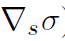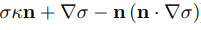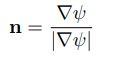# tangential surface tension

• 有人用openfoam模拟thermocapillary motion of deformable drops? Marangoni stress 需要考虑吗？直接在表面张力项加入• $\nabla_s\sigma=?$

• @东岳后两项

• 想研究一下surface tension gradient

• 这要加载动量方程中么，$\bfn$怎么封闭？

• @东岳 是的。在VOF动量方程添加。n 是后两项，我的想法是fvc::snGrad(sigma) - fvc::snGrad(alpha)*(fvc::snGrad(alpha)&fvc::snGrad (sigma))，但是编译一直不成功。

• fvc::snGrad(sigma) 这个从理论上有错误，sigma是表面张力，在不在界面的时候，是没有表面张力的。如果这么定义，回存在一个全场的值。你最好把5楼中的方程继续推导下。

• @东岳
在interfaceProperties.C中编程如下
fvc::interpolate(fvc::grad(sigma) - fvc::grad(alpha)*(fvc::grad(alpha)&fvc::grad (sigma))*mag(fvc::grad (sigma))) & mesh.Sf()
将此项添加到速度和压力方程。其中当计算压力项时发散了。